#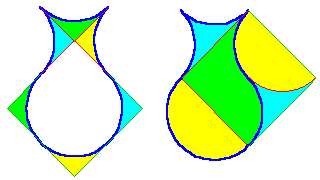Curved shapes

Of course we discard the 4 pieces dissection and keep only the less obvious 3 pieces only !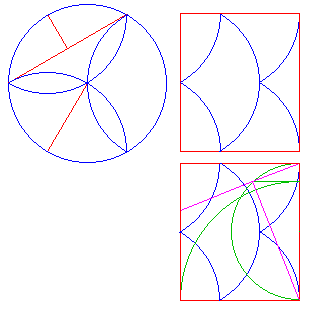The hollow circle is done in the same way, as the total length of concave arcs equals the total length of convex arcs, all being the same radius. It is then easy to get a rectangle in just a few cuts.
Then we have to dissect it into a square, by the usual techniques, and copy the cuts onto the circle.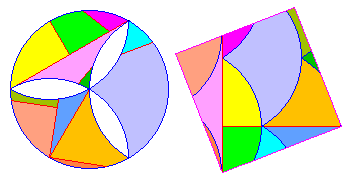The challenge is now to get less than 12 pieces !
Please note that, from another dissection, the hollow circle has the same area as the inscribed hexagon.

#### Crescent to square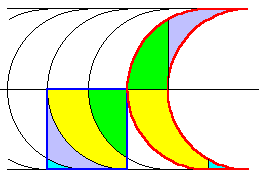We can easy duplicate the crescent to build a tape, but the width of this tape is larger than the equivallent square (diagonal) hence it is more tricky.
The dissection depends on the exact shape of the crescent. We'll consider here two shapes :
• The "Sam Loyd's" crescent, height 5×width, < 180° arcs
• The "Dudeney's" crescent, semi-circles, height = diameter = 4× width.

For dissecting into a square, the Dudeney's crescent is perfect : just overlay the square on a tape built by half crescents. We get then a 4 pieces only dissection. Easy...

The Sam Loyd's crescent is harder.
Considering the dissection of two squares 2×2 + 1×1 into one square gives the equivallent area of 5 units. Overlaying the tape of crescents and a tape of square pairs, with their own dissection (A-M-B) into one square, results then into an 8 pieces dissection.
For this dissection to work, the two tapes must be properly offset.
A minimum number of pieces is then obtained when the midline is evenly divided by squares and crescent edges. That is the crescent goes through point W.

Sorry, your browser is not Java compliant. This applet shows this :
The crescents can be shifted by dragging the yellow point, initialy at W.
We can choose the value of arc by dragging the center I, defining arc radius.
The limit is a semi-circle with diameter 5 times the crescent width, "Dudeney like".
Point M then comes exactly on a copy of the crescent (3² + 4² = 5²)
By increasing the radius, M is no more on that circle, an the piece MBP'M' has then a very small non null side MM'.
When P, or even P", comes in B, there is one less piece.
For large values of the radius, the "crescent" doesn't look a crescent anymore...
The crescent precisely defined by Sam Loyd has a radius = 26/2 times the width.
(This is for making the dissection into greek cross to work).

Gavin Theobald has found a dissection of Sam Loyd's crescent into a square in just 7 pieces.
We might wonder why this dissection has not been found sooner. May be everybody was obscured by the 5 squares...
However this dissection seems afteral natural : "just" overlay a strip of half crescents and a strip of equivallent rectangles.
The dissection of this rectangle into a square is then "as usual" with a P-slide.
Here also, we may shift the strips to get infinitely many variants.
The following applet shows this dissection. Point I defines the radius.
The yellow point shifts the crescents relative to rectangle ABCD
The initial position in the applet corresponds to Gavin Theobald's dissection of the Sam Loyd's crescent. (type R to return to this position)
The dissection in 7 pieces works as long as the cuts of the P-slide don't interfere with the crescents edges.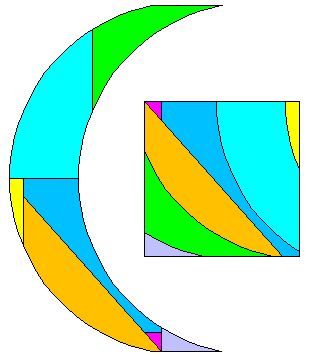Sorry, your browser is not Java compliant.
The same method works when the height is not 5×witdh (point B is draggable).
It might then be worthy ... or not.
In the case of Dudeney's crescent (h = 2R = 4d) the rectangle is even ... directly the equivallent square !
For h ≤ 4d the P-slide just vanishes, it should be done in the other direction.

####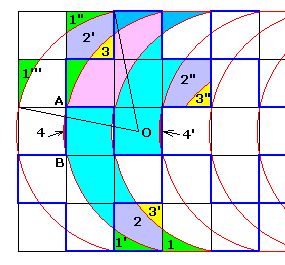Crescent into greek cross

The greek cross being made of 5 unit squares, the Sam Loyd's crescent (height = 5× width) is suitable.
The crescent should go through the grid points, his radius is then R² = 0.5² + 2.5² = 26/4 that is R = 26/2 as written above.
The construction and proof is direct on the picture.
Just note that the tip 1 of crescent equals (translation) to 1' and the square 1' 2 3' equals (translated reflection) the square 1"2'3. The piece 3 of crescent completes then this square. As 1" = 1''' (reflection at 45°), the pieces 2 and 3 of crescent fit into 2"3" of the cross (translation of the remainder of the square containing 1''').
The other pieces are obvious.

Don't forget the piece 4, little bit outside the cross, put on the other side in 4'.
That is 7 pieces.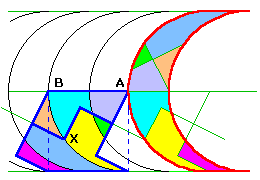The Dudeney's crescent is dissected into a greek cross by replacing the overlayed tape of square by an equivallent tape of crosses. The equivallent square is drawn as dotted lines.
To prove the dissection, we have just to prove that point X is on the circle.
That is AX = AB, which is quite obvious from the greek cross to square dissection.

####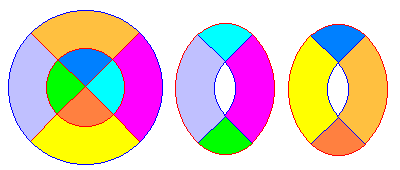Circular table

The trick is there is no concave arc in the initial disk.
A well known and (too) easy solution dissects the table into 8 pieces.
Sam Loyd in his "Cyclopedia of 5000 puzzles" gives a dissection with 6 pieces only, using the taijitu or chinese monad (Yin / Yang).
All arcs have radius R/2 and are semi or quarter circles.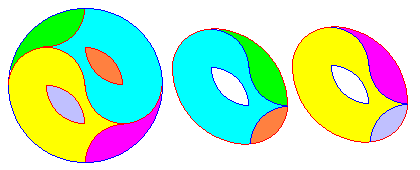There are other dissections with 6 pièces, and even 5 pieces dissections in Greg Frederickson's Web site
Also a variant in 4 pieces, with a different hole :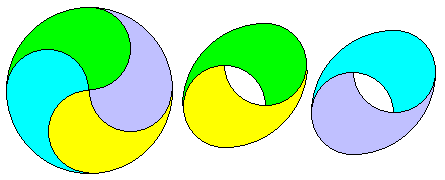####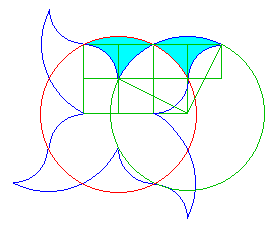Flowers

Weird shapes may result into obvious dissections ... once we have seen where to cut !
For instance a flower with 4 petals into a circle.
The grid gives the construction and the proof. No further comments.

Generalizing to a different number of petals is much harder.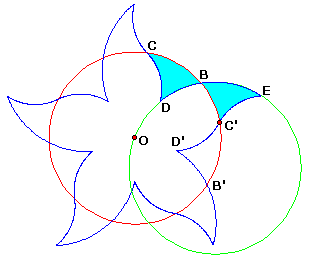Let's search for general n petals, here n = 5.

Although there might be different flower shapes (as in the 4 petals example above), we'll assume that the big circle arc goes through the flower's center, and the two small arcs are symmetric from there common point, located on the equivallent circle.
Of course the large green circle and the red equivallent circle have the same radius.
Arcs DB = BC' and BE = CB imply that arc DE = CC' = 2π/5 (2π/n in general case).
Also D' is deduced from D through a rotation by 2π/5, and C' is midpoint of D'E.
These last conditions define a unique location for D on circle ODBE, and all other points deduce.
The small arcs have any radius, just not overflowing...

Study this construction a little more with an applet allowing to vary the arc CC', really BB'. The buttons define n. The pattern is repeated 6 times (overlaying if n<6).
The n = 2 case (a digon) distorts the drawing of the arcs in the applet, but the construction is simplified in that case : D and D' come in O and the arcs are semi-circles !
Dragging B' to define arc BB' is totally free, but if not 2π/n, n integer, this doesn't result into a correct flower, petals overlap.
However the construction is 'locally' valid (for one petal).

Sorry, your browser is not Java compliant.
Let A the center of circle ODBE, then <)DAE = 2π/n, and <)DOE = DAE/2 (inscribed angles)
As <)DOD' = 2π/n also, we deduce OD' is the reflection of OD through line OE.
Midpoint C' of D'E is then the reflection of F midpoint of DE, hence the construction :
Construct the apothem a of an inscribed n-gon, a circle centered in A with radius a intersects the equivallent red circle into F, then DE _|_ AF gives DE = side of n-gon (hence DAE = 2π/n) and F midpoint of DE.
Other points are easy deduced from D and E.
Remains to prove the dissection, by prooving that the such constructed arc BC' equals the arc BD.

Let A' the diametrally opposite point to A
Hence A'DFE in line (AFA' = π/2)
Let α = <)FA'O, and of course <)A'FO = α (A'OF isosceles) and <)FOA = 2α (inscribed angle).
Let β = <)DEO, here also <)DAO = 2β
In triangle FOE, <)FOE = π - β - (π - α) = α - β
<)C'OA = <)FOA - 2<)FOE = 2α - 2(α - β) = 2β
Angles <)C'OA and <)DAO are then equal, then arcs OD and AC' also, and finally arcs BD = BO - OD and BC' = BA - AC' are equal. QED.